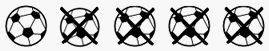Skill 3E
Using Pictures to Write a Subtraction Number Sentence

Here we want to determine if your student can look at a picture and make a number sentence from the picture. In this case, we want to determine if your student understands that "x's" over top of an object (in this case soccer balls) means that we are taking away (or subtracting the object).   Therefore, here the correct number sentence would be five soccer balls minus four soccer balls equals one soccer ball.
Problem should be answered quite quickly as this should be an easy problem to do for someone who understands that crossed-out objects means subtraction.

Look at the subtraction picture problem.
What subtraction number sentence matches the picture?4 - 5 = 1

4 + 1 = 5

5 - 4 = 1

5 + 4 = 9